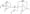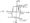# Linear IC’s and Applications August 2004

Note: i. Answer any FIVE full questions,

2. All questions carry equal marks

3. Missing data if any can be assumed suitably.

1. (a) Write the circuit of dual input balanced output differential amplifier and hence derive the expression for CMRR using h-parameter model.

(b) Design a Dual input balanced output differential amplifier with a constant current bias using diodes to satisfy the following requirements

i)    Differential voltage gain Ad — 40 ± 10

ii)    Current supplied by the constant current bias circuit = 4mA

iii) Supply voltages Vs= ±10 V

2.  (a) Explain the role of a level translator in a differential amplifier with a suitable circuit and write suitable design equations for the same.

(b) Explain the principle of frequency compensation in an OPAMP.

(c) Calculate V0 shown in the fig below.3.  (a) Draw the circuit diagram of an OPAMP mono stable multivibrator using OPAMP. With waveforms, derive an expression for output pulse width.

(b) Design a circuit to obtain the following transfer characteristics.(c)  Explain a non inverting adder with two inputs and derive an expression for output voltage. (Using one OPAMP only).

4. (a) Design a lowpass filter to meet the following specifications :

i) Butterworth approximation response

ii) Cutoff frequency = 1 kHz

iii)    Falling gain at a rate of 40 dB/dec.

(b) With a suitable diagram explain a basic logamplifier using a diode. How is temperature compensation provided for the above circuit? Hence explain the modified circuit and obtain the expression for output voltage.

5.   (a) Explain the function of a 555 using its block diagram. How can it be used as

an astable multivibrator with duty cycle less than 50%?

(b) Explain a precision type full wave rectifier with a suitable diagram.

6. a) With a neat circuit diagram, explain the working of a dual slope ADC. List its advantages.

(b) Explain the working of a 3 bit flash ADC with a neat diagram.

(c) Suppose that the digital input word for a four bit DAC changes from 0000 to 0110, calculate the DAC’s final output voltage.

7.   (a) Explain how a PLL is used to demodulate FM signal.

(b) Explain the following terms applicable to PLL.

a) Lock in range b) Capture range c) Hold in range.

(c) Design an OPAMP series regulator to meet the following specifications.

-IS db 3volts VJ> = 9 V

To — 10 to 15mA

Vz — 5.6V, Pz ~ 0’5w

8. Write short notes on any FOUR :

 (a) 3 Pin regulator (b) Analog multiplier (c) I to V converter (d) Switching regulator e) Peak detector.